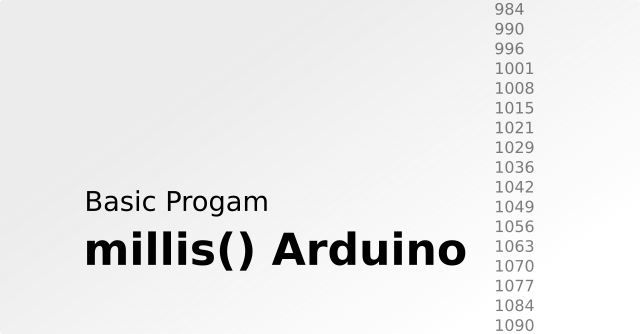# How to Use Millis on Arduino and Basic Programs

How to Use Millis Arduino and Millis Basic Program - Millis Arduino is a feature that is widely used on microcontrollers for RTOS systems. But what exactly are millis?

For timing, we usually use a time device called an RTC (Real Time Clock). Some of the commonly used RTCs such as the DS1307 and DS3231.

Apart from RTC, we can also use a timing feature that by default we can access the microcontroller, namely Millis.

## A. What is Millis?

In Arduino programming, generally we will know Delay, RTC, and Millis. All of that is used for timing.
Delay is the time delay. Delay (1000) is a delay of 1 second.

RTC is a separate timing using a specific IC which can provide complete time information

Millis is milli-second or milli-second. 1 Seconds = 1,000 milliseconds or 1 second.

When Arduino is first turned on, the millis will start counting continuously. So, millis is inactive by our program, but activates when Arduino is turned on.

How long does it take to count? Millis will count (running) starting from the numbers 0 to 4,294,967,296. If converted to days about 49 days.If we want to make a program that can interrupt for 1 second, then using delay will cause the program to stop reading every 1 second.

Unlike the millis, it will calculate the time at the same time as our Arduino program is running. If the counted time is up to 1 second, Arduino will make an interrupt.

## B. How to use Millis Arduino?

By default, the Millis value on Arduino can be called only by using the command "millis ();". If you upload the code below to Arduino, then you open the serial monitor, you will see the numbers continue to grow. These numbers are milli-second numbers.

```void setup() {
Serial.begin(9600);
}
void loop() {
Serial.println(millis());
}
```

## C. The basic program of Millis

For example, we want to display the text "Yups 1 Second" every 1 second on the Arduino Serial monitor, the algorithm is to compare the value of the previous list with the current list.

Please upload the following program to your Arduino, then please open the serial monitor. Then you will see that every 1 second (1000ms) the text "Yups 1 Second" will appear.

```const unsigned long timeVal = 1000;
unsigned long prevVal = 0;
unsigned long nowVal = 0;
void setup()
{
Serial.begin(9600);
}
void loop()
{
nowVal = millis();
if ((nowVal - prevVal) >= timeVal)
{
Serial.println("Yups 1 Second");
prevVal = nowVal;
}
}
```

That's the tutorial this time. Thank you for visiting the whis website. Hope it is useful.